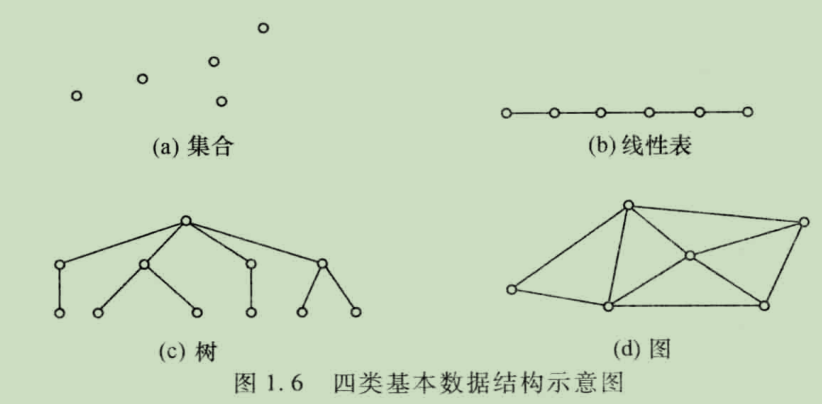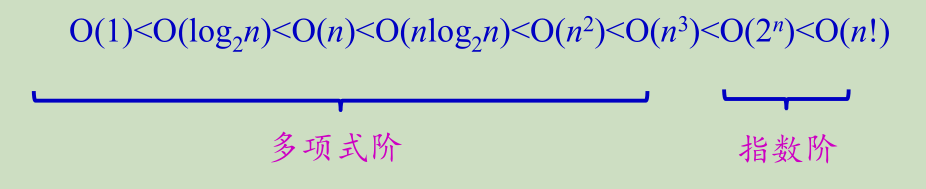# 第一章 数据结构论叙

## 1.1什么是数据结构？

### 1.1.1数据之间的关系（脑子版本）## 1.2认识一个新的数据结构

（一种抽象逻辑表示，理解为模型）

定义一个复数

InitComplex(&C)
操作结果：构造一个复数。
GetReal(C, &real)
初始条件：复数C存在。
操作结果：用real返回复数C的实部。
GetImage(C, &image)
初始条件：复数C存在。
操作结果：用image返回复数C的虚部。
OutputComplex(C)
初始条件：复数C存在。
操作结果：输出复数C的值。
初始条件：复数C1,C2存在。
操作结果：用复数C返回复数C1,C2的和。
Sub(C1,C2,&C)
初始条件：复数C1,C2存在。
操作结果：用复数C返回复数C1,C2的差。
Mul(C1,C2,&C)
初始条件：复数C1,C2存在。
操作结果：用复数C返回复数C1,C2的乘积。
Div(C1,C2,&C)
初始条件：复数C1,C2存在。
操作结果：用复数C返回复数C1除以C2的值。


## 1.3算法

• 算法的特性

有限性，确定性，可行性，输入和输入

• 算法和程序的关系

程序：使用某种计算机语言对一个算法的具体实现。

算法：重于对解决问题的方法描述，即要干啥。可用自然语言，类语言，高级语言。

• 算法设计的要求：

正确性，可读性，健壮性，高效率低储存量。

## 1.5算法分析（重点内容)

• 主要包含两个方面：正确性成本

正确性是基本的，成本就是评价算法优劣的，当然执行时间和运行所用储存空间就是判断方式。

### 1.5.1算法执行时间分析方式

#### 1.5.1.2事前估算分析方法：

##### 渐进分析

T(n) = O(f(n))表示存在一个正的常数C，使得当n≥n 0 时都满足：
|T(n)|≤C|f(n)|
– f(n)是T(n)的 上界
– 这种上界可能很多，通常取最接近的上界，即紧凑上



$T(n) = 2n^2 +2n+1 = O(n^2 )$

###### 这里描述起来相对繁琐，以以下例子来表示：
• 顺序结构的时间复杂度为O(1)
int sum = 0, n = 100;
sum = (1 + n) * n / 2;
sum = (1 + n) * n / 2;
sum = (1 + n) * n / 2;
sum = (1 + n) * n / 2;
printf(“%d”, sum);

//明显这段代码执行次数为长输，语句频度是恒定的，是常数阶
//一般来讲，莫得循环

• 线性阶 O(n)
int i;
for (i=1; i<= n; i++)
x=x+1;
//一重循环

• 平方阶 O(n2 )
for (i=1; i<= n; i++)
for (j=1;j<= n; j++)
x=x+1;  //这句叫做基本操作
//二重循环->推广到多重

• O(log n)
int count = 1；
while (count < n)
{
count = count * 2;
}



$2^x = n$

• 数据结构中常见的时间复杂度的比较关系Ps： n log2n叫二维型

#### 1.5.2算法的空间复杂度

$S(n)=O(f(n))$

###### 例子
• 若算法执行所需要的辅助空间为常数 时间复杂度为O(1)
• 举个栗子为 O(n)
for( i = 0; i < n; i++)
b[i] = a[n-i-1];
for( i = 0; i < n;i++)
a[i] = b [i];


©️2019 CSDN 皮肤主题: 游动-白 设计师: 上身试试